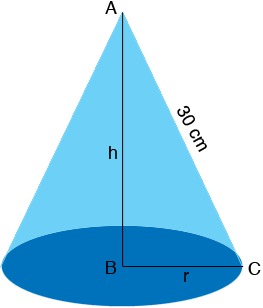SEARCH HOMEMath Central Quandaries & QueriesQuestion from Conlan, a student: Hi I am dong calculus at school and I'm stumped by this question: A cone has a slant length of 30cm. Calculate the height, h, of the cone if the volume is to be a maximum. If anyone can help me it would be greatly appreciated. thanks.Hi Conlan,

I drew a diagram of your cone with height h cm and r cm as the radius of the base.The volume of the cone in cubic centimeters is given by

$V = \frac13 \pi\; r^2 h.$

To complete the setup of this problem you need an equation relating $r$ and $h$ which you can use to eliminate $r$ from the equation for $V$ and allow you to use the calculus you know to maximize $V.$ What does Pythagoras Theorem tell you about the triangle ABC?

PennyMath Central is supported by the University of Regina and The Pacific Institute for the Mathematical Sciences.